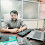# Calendar module in python

12

This module is basically used to display a calendar of the current month or range of months.

import calendar

### print(calendar.month(2014,3)) print(calendar.firstweekday()) if (calendar.isleap(2020)):    print ("The year is leap year") else :    print ("The year is not leap year") print (calendar.leapdays(1950, 2000))

print ("The calender of year 2018 is : ")
print (calendar.calendar(2018, 2, 1, 6))        # Y, W,  no. of lines per week ,  No Of columns

ASSIGNMENT using Calendar?
1)  WAP to calculate the total age in year, month, and a day where the date of birth will be entered by the user?
Solution of this program by Shiva Sir:-
from datetime import date
a = date(date.today().year, date.today().month, date.today().day)
b = date(int(input("enter year")), int(input("enter month")), int(input("enter day")))
if(a.month>b.month):
months = a.month - b.month
else:
months = b.month - a.month
years = a.year - b.year
if(a.day>b.day):
days = a.day - b.day
else:
days = b.day - a.day
print('{0} years, {1} months, {2} days'.format(years, months, days))
2)  WAP to display before the day, after day when the user enters a number of days.
3)  WAP to create STOP watch when pressings s then start, p then pause and  e then end?

Tags

1.ABHISHEK GOYAL
WAP to calculate total age in year, month, and a day where the date of birth will be entered by the user?

from datetime import date

print("##### Welcome to age calculator ######")
birth_year = int(input("Enter your year of birth: \n"))
birth_month = int(input("Enter your month of birth: \n"))
birth_day = int(input("Enter your day of birth: \n"))

current_year = date.today().year
current_month = date.today().month
current_day = date.today().day

age_year = current_year - birth_year
age_month = abs(current_month-birth_month)
age_day = abs(current_day-birth_day)

print("Your exact age is: ", age_year, "Years", age_month, "months and", age_day, "days")

1.use this program to correct ouput
from datetime import date

a = date(2021, 1, 5)
b = date(1996, 12, 13)
#print(type(a))

months = a.month - b.month
years = a.year - b.year
days = a.day - b.day

print('{0} years, {1} months, {2} days'.format(years, months, days))

2.import calendar
from datetime import date

year = int(input("Enter your birth year = "))
month = int(input("Enter your birth month in numeric form = "))
day = int(input("Enter your birth date = "))

cy = (date.today().year)
cm = (date.today().month)
cd = (date.today().day)

y = cy-year
m = cm-month
d = cd-day

if d<0 or m<0:
y =y-1
m =m+12
d = d+30

print("You are ",y,"years ",m,"months ",d,"days old")

3.WAP to calculate total age in year, month, and a day where the date of birth will be entered by the user?
import calendar
from datetime import date
year=int(input("Enter the your birth year ="))
month=int(input("Enter the your birth month ="))
day=int(input("Enter the your birth day ="))
current_yr=(date.today().year)
current_mt= (date.today().month)
current_dy=(date.today().day)
age_y=current_yr-year
age_m=current_mt-month
age_d=day-current_dy
if age_d<0 or age_m<0:
age_y=age_y-1
print("yor are ",age_y,"years",age_m,"months",age_d,"days olds")

4.#WAP to display before the day, after day when the user enters a number of days.
import calendar
a=int(input("Enter the number of week ="))
days= {1:'monday',2:'tuesday',3:'wednesday',4:'thursday',5:'friday',6:'saturday',7:'sunday'}
for i ,j in days.items():
if a==i:
print("before day =",days[a-1])
print("today = ",days[i])
print("after day = ",days[a+1])

#WAP to create STOP watch when pressings s then start, p then pause and e then end?
import time
start=raw_input("press enter to start stopwatch")
print("timer has start")
begin=time.time()
endtimer =input("press 0 to exit the stopwatch ")
print("stopwatch end")
end=time.time()

elapsed=end-begin
elapsed=int(elapsed)
print(elapsed ,"seconds")
exit(0)

5.import datetime
cy = datetime.date.today().year
cm = datetime.date.today().month
cd = datetime.date.today().day
ay=cy-by
am=abs(cm-bm)

6.WAP to calculate total age in year, month, and a day where the date of birth will be entered by the user?

import datetime
current_year = datetime.date.today().year
current_month = datetime.date.today().month
current_day = datetime.date.today().day
birth_year = int(input("enter your birth year "))
birth_month = int(input("enter your birth month "))
birth_date = int(input("enter your birth date "))
y=current_year-birth_year
m=current_month-birth_month
d=current_day-birth_date
print("your age is :" , y,"years",m,"months",d,"days")

7.n=int(input("enter a number of day"))
if (n<=7):
if (n==1):
print("TODAY IS SUNDAY NEXT DAY MONDAY BEFORE DAY SATURDAY")
elif (n==2):
print ("TODAY IS MONDAY NEXT DAY TUESDAY BEFORE DAY SUNDAY")
elif (n==3):
print ("TODAY IS TUESDAY NEXT DAY WEDNESDAY BEFORE DAY MONDAY")
elif (n==4):
print ("TODAY IS WEDNESDAY NEXT DAY THURSDAY BEFORE DAY TUESDAY")
elif (n==5):
print ("TODAY IS THURSDAY NEXT DAY FRIDAY BEFORE DAY WEDNESDAY")
elif (n==6):
print ("TODAY IS FRIDAY NEXT DAY SATURDAY BEFORE DAY THURSDAY")
else:
print("TODAY IS SATURDAY NEXT DAY SUNDAY BEFORE DAY FRIDAY")
else:
printf ("Invalid Entry")

8.from datetime import date, timedelta
s=int(input("enter days"))

current_date = date.today().isoformat()
days_before = (date.today()-timedelta(days=s)).isoformat()
days_after = (date.today()+timedelta(days=s)).isoformat()

print("\nCurrent Date: ",current_date)
print(s,"days before current date: ",days_before)
print(s,"days after current date : ",days_after)

9.from datetime import date

print("AGE calculator")

user_date=int(input("please fill day of birth : "))
user_month=int(input("please fill month of birth : "))
user_year=int(input("please fill year of birth : "))

day = date.today().day
month = date.today().month
year = date.today().year

ageday= day-user_date
agemonth= month-user_month
ageyear=year-user_year

10.from datetime import date

print("AGE calculator")

user_date=int(input("please fill day of birth : "))
user_month=int(input("please fill month of birth : "))
user_year=int(input("please fill year of birth : "))

day = date.today().day
month = date.today().month
year = date.today().year

ageday= day-user_date
agemonth= month-user_month
ageyear=year-user_year

11.from datetime import date
day,month,year=int(input("Enter day:-")),int(input("Enter Month:-")),int(input("Enter year:-"))
y,m,d=date.today().year,date.today().month,date.today().day
if m>month:
if d>day:
a=y-year
b=m-month
c=d-day
else:
a=y-year
b=m-month
c=30-(day-d)
else:
if day>d:
a=(y-year)-1
b=12-(month-m)-1
c=30-(day-d)
else:
a=(y-year)-1
b=12-(month-m)
c=d-day
print("Age is {} years {} months and {} days".format(a,b,c))